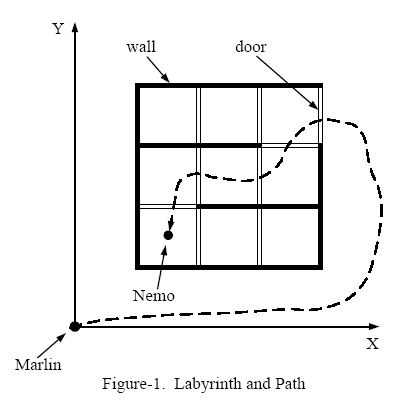POJ 2049— Finding Nemo（三维BFS）10/200

。。。同一时候也是我第一次使用  三维建图+BFS，纪念一下！

2049 算是我攻克POJ计划的第一个卡两天的难关。POJ完毕进度  10/200，为了庆贺一下。我决定回去睡个觉；

input

0 0
-100.0 -100.0

-1 -1

0

Finding Nemo
 Time Limit: 2000MS Memory Limit: 30000K Total Submissions: 7410 Accepted: 1725

Description

Nemo is a naughty boy. One day he went into the deep sea all by himself. Unfortunately, he became lost and couldn't find his way home. Therefore, he sent a signal to his father, Marlin, to ask for help.
After checking the map, Marlin found that the sea is like a labyrinth with walls and doors. All the walls are parallel to the X-axis or to the Y-axis. The thickness of the walls are assumed to be zero.
All the doors are opened on the walls and have a length of 1. Marlin cannot go through a wall unless there is a door on the wall. Because going through a door is dangerous (there may be some virulent medusas near the doors), Marlin wants to go through as few doors as he could to find Nemo.
Figure-1 shows an example of the labyrinth and the path Marlin went through to find Nemo.We assume Marlin's initial position is at (0, 0). Given the position of Nemo and the configuration of walls and doors, please write a program to calculate the minimum number of doors Marlin has to go through in order to reach Nemo.

Input

The input consists of several test cases. Each test case is started by two non-negative integers M and N. M represents the number of walls in the labyrinth and N represents the number of doors.
Then follow M lines, each containing four integers that describe a wall in the following format:
x y d t
(x, y) indicates the lower-left point of the wall, d is the direction of the wall -- 0 means it's parallel to the X-axis and 1 means that it's parallel to the Y-axis, and t gives the length of the wall.
The coordinates of two ends of any wall will be in the range of [1,199].
Then there are N lines that give the description of the doors:
x y d
x, y, d have the same meaning as the walls. As the doors have fixed length of 1, t is omitted.
The last line of each case contains two positive float numbers:
f1 f2
(f1, f2) gives the position of Nemo. And it will not lie within any wall or door.
A test case of M = -1 and N = -1 indicates the end of input, and should not be processed.

Output

For each test case, in a separate line, please output the minimum number of doors Marlin has to go through in order to rescue his son. If he can't reach Nemo, output -1.

Sample Input

```8 9
1 1 1 3
2 1 1 3
3 1 1 3
4 1 1 3
1 1 0 3
1 2 0 3
1 3 0 3
1 4 0 3
2 1 1
2 2 1
2 3 1
3 1 1
3 2 1
3 3 1
1 2 0
3 3 0
4 3 1
1.5 1.5
4 0
1 1 0 1
1 1 1 1
2 1 1 1
1 2 0 1
1.5 1.7
-1 -1```

Sample Output

```5
-1```

Source

ME             time          WA     RE     AC

1536KB      32ms         15   +   9   =  1

``````#include <iostream>
#include <stdio.h>
#include <stdlib.h>
#include <string.h>
#include <algorithm>
#define right 0
#define up    1
#define wall  3
#define door  4
#define null  0
const int N = 210;
const int Size = 999999;
const int INF = 1<<20;
using namespace std;
struct node{
int x,y,ans;
}q[Size];
int mapp[N][N];
int vis[N][N];
int n,m,result;
int mv = {{1,0},{0,-1},{0,1},{-1,0}};

void init()
{
result = 0;
memset(mapp,0,sizeof(mapp));//初始化空地
memset(vis,0,sizeof(vis));
}
void BFS(int x,int y)
{
node f,t;
int s = 0,e = 0;
f.x = x;
f.y = y;
f.ans = 0;
q[e++] = f;
vis[x][y] = 1;
result = INF;
while(s < e)
{
t = q[s++];
if(t.x ==0 || t.y ==0 || t.x > 198 || t.y > 198 ) //边界处理，可是不能跳出。仅仅能跳过，由于存在多个门的情况
{
if(result > t.ans)  //走出去之后。保存最小通过门数
result = t.ans;
continue; //注意，不要break。
}

for(int i = 0;i<4;i++)
{
f.x = t.x + mv[i];
f.y = t.y + mv[i];
// printf("asd\n");
if(i==0)
{
if(!vis[f.x][f.y] && mapp[t.x][t.y][up]!=wall)//右
{
if(mapp[t.x][t.y][up]==door)
f.ans = t.ans +1;
else
f.ans = t.ans;
vis[f.x][f.y] = 1;
q[e++] = f;
}
}
else if(i==1)
{
if(!vis[f.x][f.y] && mapp[f.x][f.y][right]!=wall) //下
{
if(mapp[f.x][f.y][right]==door)
f.ans = t.ans +1;
else
f.ans = t.ans;
vis[f.x][f.y] = 1;
q[e++] = f;
}
}
else if(i==2)
{
if(!vis[f.x][f.y] && mapp[t.x][t.y][right]!=wall)//上
{
if(mapp[t.x][t.y][right]==door)
f.ans = t.ans +1;
else
f.ans = t.ans;
vis[f.x][f.y] = 1;
q[e++] = f;
}
}
else if(i==3)
{
if(!vis[f.x][f.y] && mapp[f.x][f.y][up]!=wall)//左
{
if(mapp[f.x][f.y][up]==door)
f.ans = t.ans +1;
else
f.ans = t.ans;
vis[f.x][f.y] = 1;
q[e++] = f;
}
}
}
}
}

int main()
{
int x,y,d,t;
double Nemo_x,Nemo_y;
while(~scanf("%d%d",&m,&n))
{
if(n==-1 && m==-1)
break;
init();
for(int num_wall = 0;num_wall < m;num_wall++)
{
scanf("%d%d%d%d",&x,&y,&d,&t);
if(d)
{
for(int num = 0;num < t;num++)
mapp[x-1][y+num][up] = wall;
}
else
{
for(int num = 0;num < t;num++)
mapp[x+num][y-1][right] = wall;
}
}
for(int num_door = 0;num_door < n;num_door++)
{
scanf("%d%d%d",&x,&y,&d);
if(d)
mapp[x-1][y][up] = door;
else
mapp[x][y-1][right] = door;
}
int xx,yy;
cin>>Nemo_x>>Nemo_y;
xx = (int)(Nemo_x + 0.0001);//这里要注意 double 取整时 会有损失0.99999 会变成0
yy = (int)(Nemo_y + 0.0001);
if(n==0 && m==0)
{
printf("0\n");
continue;
}
if(xx <=0 || yy <= 0 || xx >= 199 || yy >= 199)  //后台可能出现 0,0 200+,200+。尽管不符合题目描写叙述
{
printf("0\n");

}
else
{
BFS(xx,yy);
(result==INF)?printf("-1\n"):printf("%d\n",result);
}
}
return 0;
}
``````

0条评论